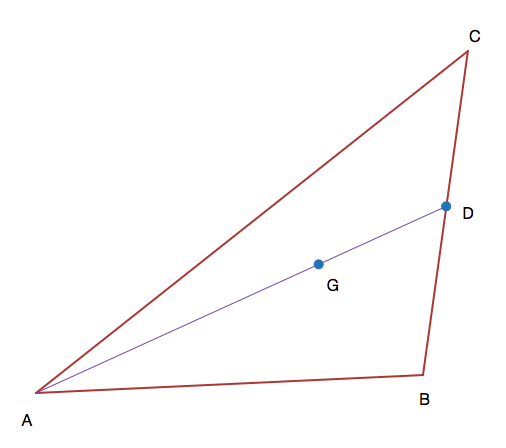# Triangle trialsTriangle $ABC$ with its centroid at $G$ has side lengths $AB=15, BC=18,AC=25$. $D$ is the midpoint of $BC$.

The length of $GD$ can be expressed as $\frac{ a \sqrt{d} } { b}$, where $a$ and $b$ are coprime positive integers and $d$ is a square-free positive integer.

Find $a + b + d + 1$.

×